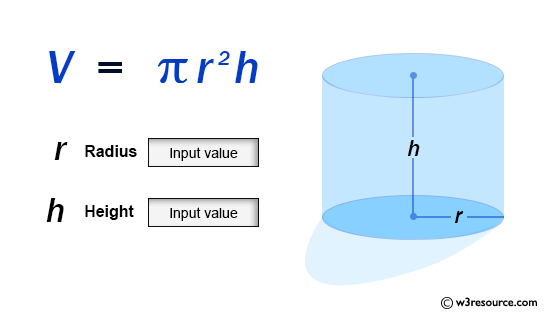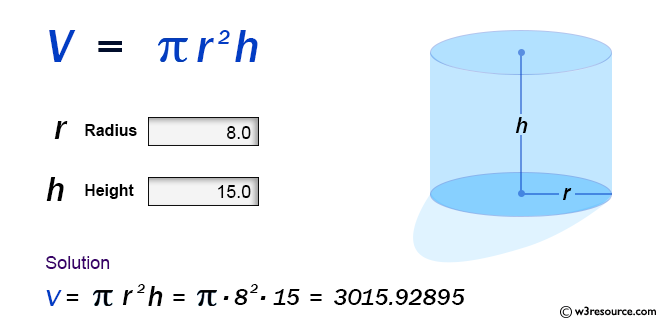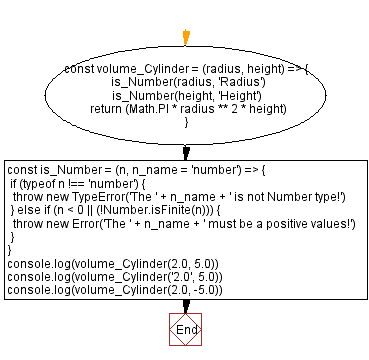# JavaScript: Volume of a Cylinder

## JavaScript Math: Exercise-60 with Solution

Write a JavaScript program to calculate the volume of a Cylinder.

From Wikipedia -
A cylinder has traditionally been a three-dimensional solid, one of the most basic of curvilinear geometric shapes. In elementary geometry, it is considered a prism with a circle as its base.
A cylinder may also be defined as an infinite curvilinear surface in various modern branches of geometry and topology. The shift in the basic meaning - solid versus surface (as in ball and sphere) - has created some ambiguity with terminology. The two concepts may be distinguished by referring to solid cylinders and cylindrical surfaces. In the literature the unadorned term cylinder could refer to either of these or to an even more specialized object, the right circular cylinder.

Sample Data:Sample Solution:

HTML Code:

``````<!DOCTYPE html>
<html>
<meta charset="utf-8">
<title>JavaScript function to Volume of a Cylinder</title>
<body>

</body>
</html>
```
```

JavaScript Code:

``````const volume_Cylinder = (radius, height) => {
is_Number(height, 'Height')
return (Math.PI * radius ** 2 * height)
}

const is_Number = (n, n_name = 'number') => {
if (typeof n !== 'number') {
throw new TypeError('The ' + n_name + ' is not Number type!')
} else if (n < 0 || (!Number.isFinite(n))) {
throw new Error('The ' + n_name + ' must be a positive values!')
}
}

console.log(volume_Cylinder(2.0, 5.0))
console.log(volume_Cylinder('2.0', 5.0))
console.log(volume_Cylinder(2.0, -5.0))
```
```

Sample Output:

```62.83185307179586
-----------------------------------------------------
Uncaught TypeError: The Radius is not Number type!
at https://cdpn.io/cpe/boomboom/pen.js?key=pen.js-1a04af30-dc8a-5819-1580-ea8dd4385b7e:9
--------------------------------------------------------------------------------------------
Uncaught Error: The Height must be a positive values!
at https://cdpn.io/cpe/boomboom/pen.js?key=pen.js-0233eca2-a783-da3b-2f4a-b77720178072:11
```

Flowchart:Live Demo:

See the Pen javascript-math-exercise-60 by w3resource (@w3resource) on CodePen.

Improve this sample solution and post your code through Disqus

Previous: Volume of a Pyramid.
Next: Volume of a Triangular Prism.

What is the difficulty level of this exercise?

Test your Programming skills with w3resource's quiz.

﻿